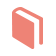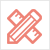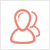﻿ 更多范文-如何选择美国的金融产品分析--美国Assignment代写范文 -51Due留学教育

#### 服务承诺资金托管原创保证实力保障24小时客服使命必达

#### 关于我们

51Due提供Essay，Paper，Report，Assignment等学科作业的代写与辅导，同时涵盖Personal Statement，转学申请等留学文书代写。51Due将让你达成学业目标51Due将让你达成学业目标51Due将让你达成学业目标51Due将让你达成学业目标

#### 名企实习积累工作经验多元化文化交流专业实操技能建立人际资源圈

# 如何选择美国的金融产品分析--美国Assignment代写范文

2017-02-10 来源: 51Due教员组 类别: 更多范文American options are financial derivatives, an instrument whose value is derived from an underlying asset, usually a stock. Black and Scholes (1973) described an option as: 'a security giving the right to buy or sell an asset, subject to certain conditions, within a specified period of time'.

The main question of this dissertation is how American options can be valued. The option value is only known with certainty when the option is exercised, either at maturity or not. When the owner decides to exercise the option or it is the option maturity time, it is possible to determine the price of the option as the strike will be exchanged by the asset in the case that the conditions are favourable for the owner of the option. When the one buys the option, she does not know what will be the future price of the underlying asset, and assuming it follows a random process it is hard to put a price on such contract without knowing what will be the price change. This non linear feature of the option makes calculating the price to pay for such contracts a challenging process and has been the focus of a large number of financial studies and publications.

This dissertation deals with the most popular methods for pricing American options and their implementation in MatLab?, including a graphic user interface.

The methods studied include the Black and Scholes (1973) European option pricing as the starting point, followed by the Barone Adesi and Whaley (1987) analytical approximation. Then the binomial and trinomial lattice methods presented in Cox, Ross and Rubinstein (1979) are considered also as the Finite difference approximations models AAA. The most sophisticated method is the Least Squares Monte Carlo simulation presented in Longstaff and Schwartz (2001).

The analysis of the different option pricing methods in this dissertation follow most of the assumptions made by Black and Scholes (1973), the short term interest rate and the dividend are assumed to be known and constant, the underlying stock follows a log normal distributed geometric Brownian motion, the markets are frictionless and finally it exists the possibility of forming a riskless portfolio, consisting of the option and underlying stock.

The dissertation is organised as follows: a brief literature survey is provided in the next Chapter. The analytical approximation method and the numerical methods used are described on Chapter 3 and their implementation in Matlab environment is given in chapter 4. Numerical results are given in Chapter 5. The conclusion and future developments are presented in Chapter 6.

Chapter 2 provides a survey of some of the most relevant publications in American Option Pricing, with focus on analytical approximations, lattice and finite difference methods, more precisely, binomial and trinomial trees, explicit, implicit and Crank

Nicolson Scheme, and also on Monte Carlo Simulation.

Chapter 3 provides a description of the methods used, their advantages, disadvantages and limitations. Here the required equations will be derived and the solution for the pricing of American options will be provided.

Chapter 4 focus on the algorithms used and their implementation on the MatLab environment, also as the procedures for the development of the GUI for easier user interface.

On Chapter 5 results and their comparison are shown for the different methods used, with the required figures to support the numerical answers.

In the final chapter the dissertation is concluded and a summary of the findings is provided, also as with further work on this subject.

Black and Scholes (1973) and Merton (1973) developed the first analytical closed form solution for the pricing of European type options and certain types of American options, such as American call options on non dividend paying stocks. 'The option pricing model developed by Black and Scholes and extended by Merton gives rise to partial differential equations governing the value of an option' Schwartz (1976).

Black and Scholes (1973) develop their model on the basis of the no arbitrage theory, 'If options are correctly priced in the market, it should not be possible to make sure profits by creating portfolios of long and short positions in options and their underlying stocks' Black and Scholes (1973).

The Black and Scholes (1973) model valued European options on non dividend paying stocks, and with a number of quite restrictive assumptions, constant and known interest rates, the markets are frictionless with no transaction costs and penalties for short selling. The Black and Scholes (1973) model also assumes that the underlying stocks follow a random walk. Due to all this assumptions the pricing model Black and Scholes (1973) proposed was of easy use, and there is only the need to input the required values on the proposed pricing equation. The model they have proposed does not take into consideration early exercise of the option so it is inaccurate for pricing American Options.

One of the most popular analytical approximation models that starts from the Black and Scholes (1973) model and adjusts it to consider the scenario of early exercise strategies is the work by Baron Adesi and Whaley (1987) which was based on the paper by MacMillan (1986).

Baron Adesi and Whaley (1987) consider that the Black and Scholes (1973) partial differential equation must apply to the early exercise premium as this is just the difference between the American and the European option prices, which are also priced by the same partial differential equation. After some transformation they end with an easily solvable through an interactive process second order differential equation.

When closed form solutions, like the Black and Scholes (1973) valuation model cannot be derived, numerical methods must be developed. These are computational methods where the values for the underlying assets are modelled up to maturity and the price of the options is derived from them. In the case of American options this is a complex process, as the modelled price changes may have to be adjusted to include dividend payments and the derivation of the option price must also include the possibility of early exercise.

Cox, Ross and Rubinstein (1979) developed a simple discrete time lattice model to deal with the complexity of option valuation, as they considered the methods of Black and Scholes (1973) 'quite advanced and have tended to obscure the underlying economics' Cos, Ross and Rubinstein (1979). The use of lattice models such as the one by Cox, Ross and Rubinstein (1979) is the simplicity of its application.

The most significant drawback of the Cox, Ross and Rubinstein (1979) model, is to increase its accuracy the number of time intervals must increase, in order to approach a continuous time model, which will significantly increase the computational time, needed for processing the entire tree in order to derive the option value.

Others such as Hull and White (1988), (1993) and Trigeorgis (1991) have extended the model of Cox, Ross and Rubinstein (1979).

Hull and White (1988) present a study of the use of lattice models for underlying assets with known dividends instead of known divided yields. They also consider the use of a control variate to price a option numerically, by a the lattice model, using the price of a similar option calculated analytically. While Trigeorgis (1991) proposes 'a log transformed variation of binomial option pricing designed to overcome problems of consistency, stability and efficiency encountered in the Cox, Ross and Rubinstein (1979)' focusing on the pricing of exotic options. Hull and White (1993) also present an application of binomial and trinomial procedures for exotic path dependent options, where they developed a model faster than Monte Carlo simulation and faster than other numerical methods.

Usually the analytical procedures are applicable to simple payoffs of the American Options, but in the cases where this is not possible numerical solutions must be developed. Geske and Shastri (1985) give a detailed comparison of the lattice methods to the different numerical methods, finite difference methods and other simulation methods.

The model proposed by Brennan and Schwartz (1978) for valuing options was the first approach that used the finite difference method. This approach was used due to the fact that most of the times an analytical solution for the option pricing problem does not exist. The finite difference method uses the heat equation derived from the Black and Sholes PDE to obtain an approximation of the option price. Courtadon (1998) goes further to reduce the approximation error of the Brennan and Schwartz (1978) model but only applies his findings only to simple option pay offs.

Geske and Shastri (1985) give a good description of the finite difference method: 'The finite difference technique analyze the partial differential equation (...) by using discrete estimates of the changes in the options value for small changes in time or the underlying stock price to form equations as approximations to the continuous partial derivatives.' Usually the approximations is done using forward, backward or central difference theorem, which respectively result in the explicit, implicit and Crank Nicolson schemes, the procedure used in this study will be shown further in the paper.

51due留学教育原创版权郑重声明：原创留学生作业代写范文源自编辑创作，未经官方许可，网站谢绝转载。对于侵权行为，未经同意的情况下，51Due有权追究法律责任。51due有着最专业的论文代写、paper代写、essay代写服务、matlab代写、peaking代写。

51due为留学生提供最好的统计作业代写服务，亲们可以进入主页了解和获取更多assignment代写范文 提供美国作业代写服务，详情可以咨询我们的客服QQ:800020041哟。-xz